# How To Copy a Formula Down a Column in Google Sheets

You can use Google Sheets for anything from household budgeting to managing a business. Sheets also makes short work of accounts, invoicing, and billing. One way it helps out is when formulas, and that’s the subject of today’s tutorial. This article shows you how to copy a formula down an entire column in Google Sheets to help you save time and frustration.## Help with Google Sheets Formulas

Formulas are the math behind the spreadsheet. Using particular expressions, you tell the sheet what to do with the data you enter into specific cells to generate the desired result. The task can be as simple as adding two cells to create a total and collate the averages over thousands of different cells. Regardless of the size and scope of the calculation, the core formula usually remains the same.

## How To Use Formulas In Google Sheets

Formulas are relatively straightforward, even if you are not a math geek. Google Sheets uses logical expressions to deliver results depending on the criteria you enter. You can see the formula in the actual cell containing said formula or within the formula bar at the top of the Sheet (denoted by FX). Here’s how to enter a formula in Google sheets.

1. Double click on the cell where you want your formula, and then type “=” without quotes, followed by the formula.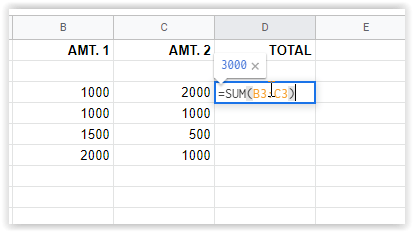2. Press Enter to save formula or click on another cell. The results will appear in the cell while the formula will show in the “fx” box above.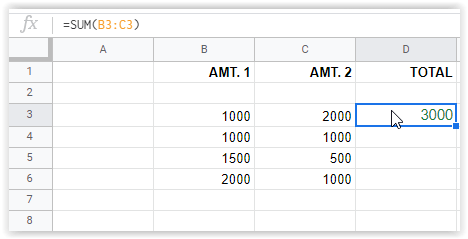In the image example above, the formula entered in cell D3 is shown in the “fx” box at the top while the value appears in the cell. The example above adds cells B3 and C3, forming a sum. It is a simple formula, but it gives you an idea of how they work.

Formulas can become complicated, advanced statements capable of functions like sorting, highlighting specific cells based on specified criteria, combining various mathematics for specific cell combinations, and much more.

## Copy a Formula Down an Entire Column in Google Sheets

To copy calculations down an entire column in Google Sheets, you have a few options, depending on the formula. You’ll understand that better when you get to option #3. The easiest method is to grab the fill handle and slide down to your last cell. However, longer sheets work best by simply double-clicking the handle. You can also use the top cell to initiate a formula replication process that flows down your entire column. Here are the details on all three options.

### Option #1: Dragging The Top Cell to Replicate Formulas

1. Highlight the first cell in your column that includes the formula, then select the fill handle (small blue box) in the cell’s bottom-right section. The cursor turns into a crosshair when positioned correctly.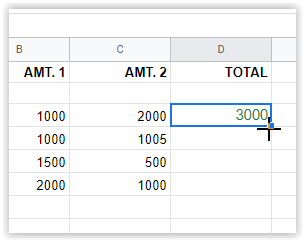2. Drag the crosshair down to the last desired cell that will use the specified formula. Google Sheets will automatically populate the correct formula for each row.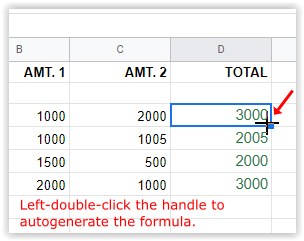The above process uses row #3’s formula [=SUM(B3+C3)] to autopopulate all other selected rows within the column [=SUM(B4+C4)], [=SUM(B5+C5)], etc.

Note: Option #1 will insert “0” in a row where no data is present. You’ll have to delete the contents of that cell if you want it blank.

### Option #2: Double-Click the Top Cell to Replicate The Formula Down The Column

1. Select the first cell in the column that includes the formula, then hover over the fill handle in the bottom-right corner. DO NOT CLICK YET.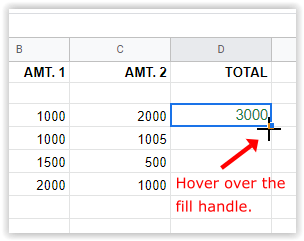2. Double-click the left mouse button while on the fill handle. This process will autogenerate the formula down to the last filled cell in the column.Note: Option #2 will stop inserting formulas when it reaches a blank row down the column. Copy the first cell, paste it in the next filled cell of the column, and replicate the steps above.

### Option #3: Use an Array Formula to Replicate Calculations Down The Column

The last method to duplicate a formula down a column in Google Sheets is to use the “ArrayFormula” function. Be sure to type the correct ranges into the formula.

#### Google ARRAYFORMULA Range Examples to Replicate Formulas in a Column

`=ArrayFormula(B3:B6+C3:C6)`
The above example uses the “addition” formula (B3+C3), but it adds a range that goes down to cells B6 and C6. Google Sheets uses (B3+C3) and replicates it down the column (B4+C4), (B5+C5), and (B6+C6).

`=ARRAYFORMULA(IF(ISBLANK(B3:B+C3:C),"",IF(B3:B+C3:C=0,"",(B3:B+C3:C))))`
The above example calculates the same totals as the previous formula, except it replaces the “0” in cells with no characters so that it appears empty. The ISBLANK part ignores blank cells, and the characters inserted inside “” are what Google Sheets places into the empty cells, which is set as nothing.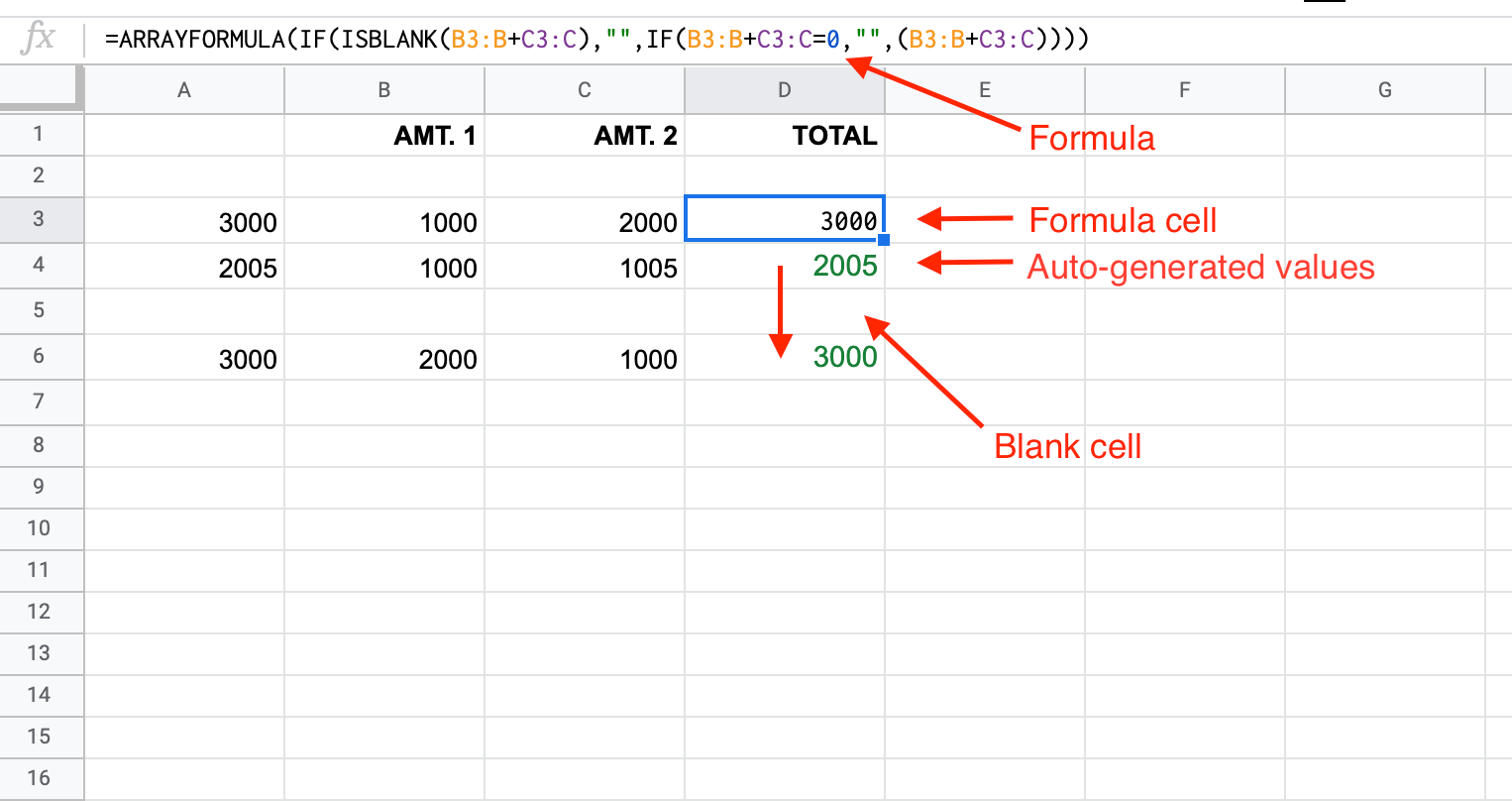Note: Option #3 will auto-populate the formula in each cell of the column based on your specified range. If any blank cells exist in the range, it will insert “0” in the cell, unless you add the “ISBLANK” and “=0” formulas, as shown above.

All cells become undeletable unless you clear the array formula in the top cell and choose another method. If you try to add a number in a cell within the array, the formula cell will display “#REF!” and all cells below it become blank except the one you changed. Delete does nothing to the cells within the array.

## Copying a Google Sheet to Apply New Formulas

A lot of data can be contained within a sheet, here’s how to copy one to test out new formulas without having to worry.

1. First, open the sheet you want to duplicate.
2. Next, right-click and select Duplicate.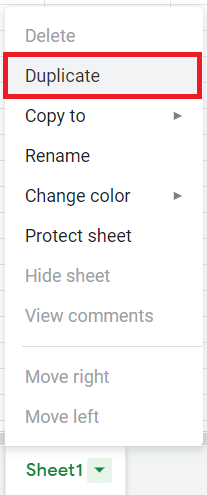3. A new sheet will be created with the name of your file with Copy of in front of it.
4. Use this sheet to test out new formulas on real-world data, etc. Make sure to have multiple copies of any important data.

In closing, the methods used in Google Sheets to replicate formulas in a column is not that complicated, as long as you understand the formula strings/arguments used. No matter which option suits you best, test it out first in a small sheet, then copy it over. It is also best to run a second test on a genuine copy of your original sheet before officially implementing the formulas, mainly because you have a lot of data that could get changed for the worse.

## 2 thoughts on “How To Copy a Formula Down a Column in Google Sheets”nonoyer biznez says:
You might want to update this. The instructions for highlighting the first cell with the formula then going to the last in the column no longer works.Steve Larner says:
Thank you. The article was recently updated with content and images to reflect accuracy.

Disclaimer: Some pages on this site may include an affiliate link. This does not effect our editorial in any way.

Todays Highlights